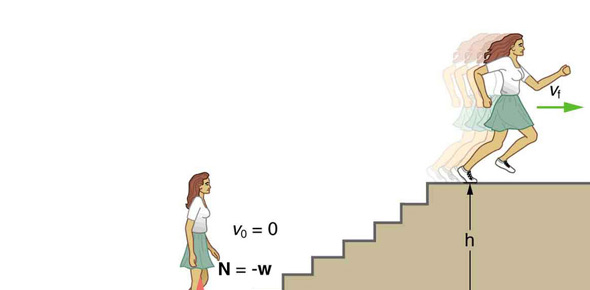# Work Power Energy IGCSE

15 Questions | Total Attempts: 1387SettingsThis quiz includes the topics on Work, Energy and Power. The quiz is consisting of 15 multiple-choice items, which are all conceptual. Time limit for the quiz is 15 minutes. Good luck.

• 1.
Which of the following is not a unit for power?
• A.

Joule – second

• B.

Watt

• C.

Newton-meter per second

• D.

Horsepower

• 2.
Car A has a mass of 1000 kg and a speed of 60 km/h, and car B has a mass of 2000 kg and a speed of 30 km/h.  The kinetic energy of car A is _____.
• A.

Half that of car B

• B.

Equal that of car B

• C.

Twice that of car B

• D.

Four times that of car B

• 3.
If an object has kinetic energy, then it also must have _____.
• A.

Speed

• B.

Impulse

• C.

Acceleration

• D.

Force

• 4.
A feather and a coin are dropped in the air.  Each falls with equal _____.
• A.

Momenta

• B.

Kinetic energies

• C.

Potential energy

• D.

None of the above

• 5.
When a bullet is fired from a rifle, the force on the rifle is equal to the force on the bullet.  However, the energy of the bullet is greater than the energy of the recoiling rifle because the _______.
• A.

Force on the bullet acts for a longer distance

• B.

Bullet's momentum is greater than that of the rifle

• C.

Force on the bullet acts for a longer time

• D.

The impulse of the bullet is more

• 6.
An object that has kinetic energy must be _____.
• A.

Moving.

• B.

Falling.

• C.

At an elevated position.

• D.

At rest.

• 7.
An object that has potential energy has this energy because of its _____.
• A.

Speed.

• B.

Acceleration.

• C.

Momentum.

• D.

Location.

• 8.
An object is raised above the ground gaining a certain amount of potential energy.  If the same object is raised twice as high it gains _____.
• A.

Four times as much potential energy.

• B.

Twice as much potential energy.

• C.

Half as much potential energy

• D.

Neither of these.

• 9.
When an object is lifted 10 meters, it gains a certain amount of potential energy. If the same object is lifted 20 meters, its potential energy is ______.
• A.

Less.

• B.

The same

• C.

Twice as much.

• D.

Four times as much

• 10.
Two identical arrows, one with twice the kinetic energy of the other, are fired into a hay bale.  The faster arrow will penetrate _____.
• A.

The same distance as the slower arrow.

• B.

Twice as far as the slower arrow.

• C.

Four times as far as the slower arrow.

• D.

More than four times as far as the slower arrow.

• 11.
A ball is projected into the air with 100 J of kinetic energy which is transformed to gravitational potential energy at the top of its trajectory.  When it returns to its original level after encountering air resistance, its kinetic energy is _____.
• A.

Less than 100 J.

• B.

More than 100 J.

• C.

100 J.

• D.

Not enough information given.

• 12.
Strictly speaking, a car will burn more gasoline if the car radio is turned on.  This statement is _____.
• A.

Totally false.

• B.

True only if the car's engine is running.

• C.

True only if the car's engine is stopped.

• D.

Always true.

• 13.
Two identical arrows, one with twice the speed of the other, are fired into a hay bale.  The faster arrow will penetrate _____.
• A.

The same distance as the slower arrow.

• B.

Twice as far as the slower arrow.

• C.

Four times as far as the slower arrow.

• D.

More than four times as far as the slower arrow.

• 14.
How many Joules of energy are in one kilowatt-hour?
• A.

1 J

• B.

60 J

• C.

60 kilojoules

• D.

3.6 megajoules

• 15.
A feather and a coin dropped in a vacuum fall with equal _____.
• A.

Forces

• B.

Momenta

• C.

Accelerations

• D.

Kinetic energies

Related TopicsBack to top